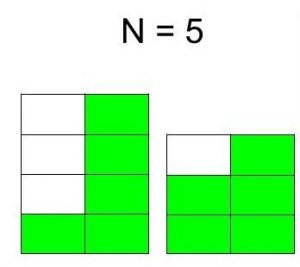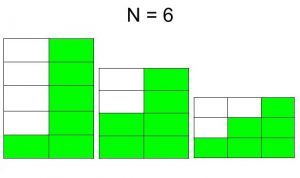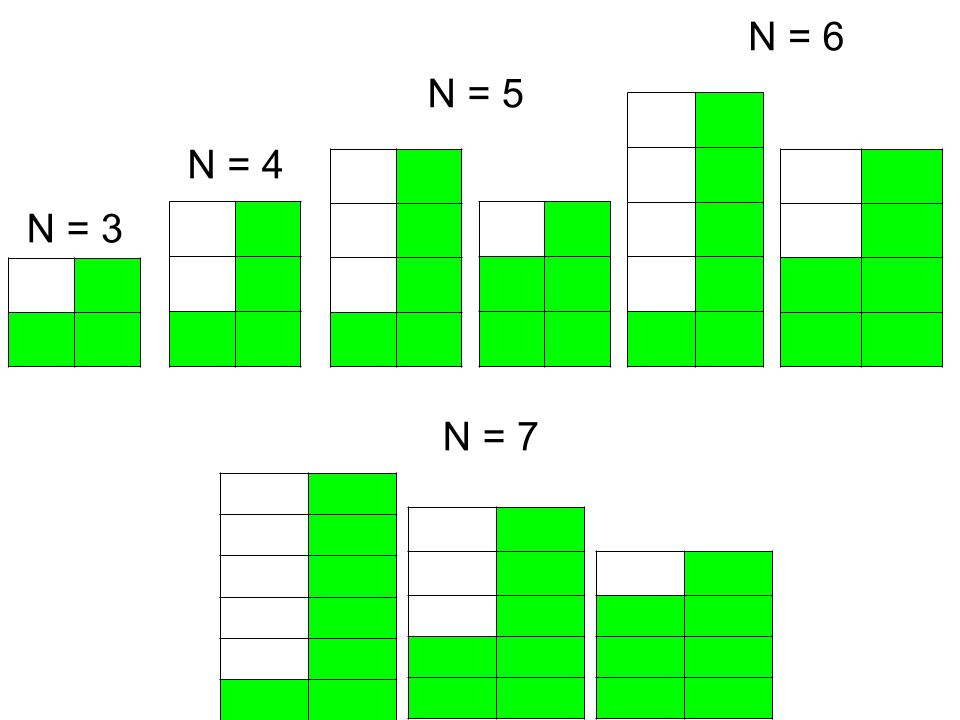# Total number of different staircase that can made from N boxes

Given N boxes each of unit dimension i.e. (1×1). The task is to find the total number of different staircases that can be made from those boxes with the following rules:

• Staircase must be in strictly descending order.
• Each staircase contains at least two steps. (Total steps is equal to the breadth of the staircase.)

Examples:

Input : N = 5
Output : 2
The two staircase are following :Input : N = 6
Output : 3
The three staircase are following :## Recommended: Please try your approach on {IDE} first, before moving on to the solution.

If we consider total steps = 2, we can observe the fact that the number of staircase is incremented by 1 if N is incremented by 2. We can illustrate the above thing from the following image :Now, if total steps is greater than 2 (assume, total steps = K) then we can take this thing as first create a base (base requires boxes equal to the total steps) for staircase and put another staircase on it of steps size K and K – 1 having boxes N – K. (because K boxes already used to create base). Thus, we can solve this problem using bottom-up dynamic programming.

Below is the implementation of the above approach:

## C++

 `// C++ program to find the total number of ` `// different staircase that can made ` `// from N boxes ` `#include ` `using` `namespace` `std; ` ` `  `// Function to find the total number of ` `// different staircase that can made ` `// from N boxes ` `int` `countStaircases(``int` `N) ` `{ ` `    ``// DP table, there are two states. ` `    ``// First describes the number of boxes ` `    ``// and second describes the step ` `    ``int` `memo[N + 5][N + 5]; ` ` `  `    ``// Initilize all the elements of ` `    ``// the table to zero ` `    ``for` `(``int` `i = 0; i <= N; i++) { ` `        ``for` `(``int` `j = 0; j <= N; j++) { ` `            ``memo[i][j] = 0; ` `        ``} ` `    ``} ` ` `  `    ``// Base case ` `    ``memo = memo = 1; ` ` `  `    ``for` `(``int` `i = 5; i <= N; i++) { ` `        ``for` `(``int` `j = 2; j <= i; j++) { ` ` `  `            ``// When step is equal to 2 ` `            ``if` `(j == 2) { ` `                ``memo[i][j] = memo[i - j][j] + 1; ` `            ``} ` ` `  `            ``// When step is greater than 2 ` `            ``else` `{ ` `                ``memo[i][j] = memo[i - j][j] +  ` `                             ``memo[i - j][j - 1]; ` `            ``} ` `        ``} ` `    ``} ` ` `  `    ``// Count the total staircase ` `    ``// from all the steps ` `    ``int` `answer = 0; ` `    ``for` `(``int` `i = 1; i <= N; i++)  ` `        ``answer = answer + memo[N][i];     ` ` `  `    ``return` `answer; ` `} ` ` `  `// Driver Code ` `int` `main() ` `{ ` `    ``int` `N = 7; ` ` `  `    ``cout << countStaircases(N); ` ` `  `    ``return` `0; ` `} `

## Java

 `// Java program to find the total number of ` `// different staircase that can made ` `// from N boxes ` ` `  `import` `java.util.*; ` ` `  `class` `GFG ` `{ ` `        ``// Function to find the total number of ` `        ``// different staircase that can made ` `        ``// from N boxes ` `        ``static` `int` `countStaircases(``int` `N) ` `        ``{ ` `            ``// DP table, there are two states. ` `            ``// First describes the number of boxes ` `            ``// and second describes the step ` `            ``int` `[][] memo=``new` `int``[N + ``5``][N + ``5``]; ` `         `  `            ``// Initilize all the elements of ` `            ``// the table to zero ` `            ``for` `(``int` `i = ``0``; i <= N; i++) { ` `                ``for` `(``int` `j = ``0``; j <= N; j++) { ` `                    ``memo[i][j] = ``0``; ` `                ``} ` `            ``} ` `         `  `            ``// Base case ` `            ``memo[``3``][``2``] = memo[``4``][``2``] = ``1``; ` `         `  `            ``for` `(``int` `i = ``5``; i <= N; i++) { ` `                ``for` `(``int` `j = ``2``; j <= i; j++) { ` `         `  `                    ``// When step is equal to 2 ` `                    ``if` `(j == ``2``) { ` `                        ``memo[i][j] = memo[i - j][j] + ``1``; ` `                    ``} ` `         `  `                    ``// When step is greater than 2 ` `                    ``else` `{ ` `                        ``memo[i][j] = memo[i - j][j] +  ` `                                    ``memo[i - j][j - ``1``]; ` `                    ``} ` `                ``} ` `            ``} ` `         `  `            ``// Count the total staircase ` `            ``// from all the steps ` `            ``int` `answer = ``0``; ` `            ``for` `(``int` `i = ``1``; i <= N; i++)  ` `                ``answer = answer + memo[N][i];  ` `         `  `            ``return` `answer; ` `        ``} ` `         `  `        ``// Driver Code ` `        ``public` `static` `void` `main(String [] args) ` `        ``{ ` `            ``int` `N = ``7``; ` `         `  `            ``System.out.println(countStaircases(N)); ` `         `  `             `  `        ``} ` ` `  `} ` ` `  `// This code is contributed  ` `// by ihritik `

## Python 3

 `# Python 3 program to find the total  ` `# number of different staircase that  ` `# can made from N boxes ` ` `  `# Function to find the total number  ` `# of different staircase that can  ` `# made from N boxes ` `def` `countStaircases(N): ` ` `  `    ``# DP table, there are two states. ` `    ``# First describes the number of boxes ` `    ``# and second describes the step ` `    ``memo ``=` `[[``0` `for` `x ``in` `range``(N ``+` `5``)] ` `               ``for` `y ``in` `range``(N ``+` `5``)] ` ` `  `    ``# Initilize all the elements of ` `    ``# the table to zero ` `    ``for` `i ``in` `range``(N ``+` `1``): ` `        ``for` `j ``in` `range` `(N ``+` `1``): ` `            ``memo[i][j] ``=` `0` `         `  `    ``# Base case ` `    ``memo[``3``][``2``] ``=` `memo[``4``][``2``] ``=` `1` ` `  `    ``for` `i ``in` `range` `(``5``, N ``+` `1``) : ` `        ``for` `j ``in` `range` `(``2``, i ``+` `1``) : ` ` `  `            ``# When step is equal to 2 ` `            ``if` `(j ``=``=` `2``) : ` `                ``memo[i][j] ``=` `memo[i ``-` `j][j] ``+` `1` `             `  `            ``# When step is greater than 2 ` `            ``else` `: ` `                ``memo[i][j] ``=` `(memo[i ``-` `j][j] ``+`  `                              ``memo[i ``-` `j][j ``-` `1``]) ` `     `  `    ``# Count the total staircase ` `    ``# from all the steps ` `    ``answer ``=` `0` `    ``for` `i ``in` `range` `(``1``, N ``+` `1``): ` `        ``answer ``=` `answer ``+` `memo[N][i]  ` ` `  `    ``return` `answer ` ` `  `# Driver Code ` `if` `__name__ ``=``=` `"__main__"``: ` ` `  `    ``N ``=` `7` ` `  `    ``print` `(countStaircases(N)) ` ` `  `# This code is contributed ` `# by ChitraNayal `

## C#

 `// C# program to find the total number  ` `// of different staircase that can made ` `// from N boxes ` `using` `System; ` ` `  `class` `GFG ` `{ ` `     `  `// Function to find the total number  ` `// of different staircase that can  ` `// made from N boxes ` `static` `int` `countStaircases(``int` `N) ` `{ ` `    ``// DP table, there are two states. ` `    ``// First describes the number of boxes ` `    ``// and second describes the step ` `    ``int` `[,] memo = ``new` `int``[N + 5, N + 5]; ` ` `  `    ``// Initilize all the elements  ` `    ``// of the table to zero ` `    ``for` `(``int` `i = 0; i <= N; i++)  ` `    ``{ ` `        ``for` `(``int` `j = 0; j <= N; j++) ` `        ``{ ` `            ``memo[i, j] = 0; ` `        ``} ` `    ``} ` ` `  `    ``// Base case ` `    ``memo[3, 2] = memo[4, 2] = 1; ` ` `  `    ``for` `(``int` `i = 5; i <= N; i++) ` `    ``{ ` `        ``for` `(``int` `j = 2; j <= i; j++)  ` `        ``{ ` ` `  `            ``// When step is equal to 2 ` `            ``if` `(j == 2)  ` `            ``{ ` `                ``memo[i, j] = memo[i - j, j] + 1; ` `            ``} ` ` `  `            ``// When step is greater than 2 ` `            ``else`  `            ``{ ` `                ``memo[i, j] = memo[i - j, j] +  ` `                             ``memo[i - j, j - 1]; ` `            ``} ` `        ``} ` `    ``} ` ` `  `    ``// Count the total staircase ` `    ``// from all the steps ` `    ``int` `answer = 0; ` `    ``for` `(``int` `i = 1; i <= N; i++)  ` `        ``answer = answer + memo[N, i];  ` ` `  `    ``return` `answer; ` `} ` ` `  `// Driver Code ` `public` `static` `void` `Main() ` `{ ` `    ``int` `N = 7; ` ` `  `    ``Console.WriteLine(countStaircases(N)); ` `} ` `} ` ` `  `// This code is contributed  ` `// by Subhadeep `

## PHP

 ` `

Output:

```4
```

Time Complexity: O().

Attention reader! Don’t stop learning now. Get hold of all the important DSA concepts with the DSA Self Paced Course at a student-friendly price and become industry ready.

My Personal Notes arrow_drop_upCheck out this Author's contributed articles.

If you like GeeksforGeeks and would like to contribute, you can also write an article using contribute.geeksforgeeks.org or mail your article to contribute@geeksforgeeks.org. See your article appearing on the GeeksforGeeks main page and help other Geeks.

Please Improve this article if you find anything incorrect by clicking on the "Improve Article" button below.